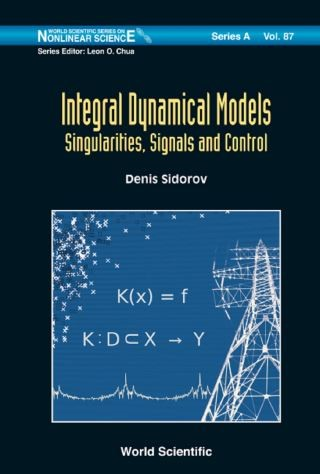## Integral Dynamical Models: Singularities, Signals And Control• Description
• Author
• Info
• Reviews

### Description

This volume provides a broad introduction to nonlinear integral dynamical models and new classes of evolutionary integral equations. It may be used as an advanced textbook by postgraduate students to study integral dynamical models and their applications in machine learning, electrical and electronic engineering, operations research and image analysis.Contents:

• Introduction and Overview
• Volterra Models of Evolving Dynamical Systems:
• Volterra Equations of the First Kind with Piecewise Continuous Kernels
• Volterra Matrix Equation of the First Kind with Piecewise Continuous Kernels
• Volterra Operator Equations of the First Kind with Piecewise Continuous Kernels
• Generalized Solutions to the Volterra Equations with Piecewise Continuous Kernels and Sources
• Nonlinear Models, Singularities and Control:
• Nonlinear Hammerstein Integral Equations
• Nonlinear Volterra Operator Equations with Non-invertible Operator
• Nonlinear Differential Equations Near Branching Points
• Convex Majorants Method in the Theory of Nonlinear Volterra Equations
• Generalized Solutions to Nonlinear Volterra Equations of the First Kind
• On Impulse Control of Nonlinear Dynamical Systems Based on the Volterra Series
• Integral Models Applications:
• The Volterra Models Applications
• Suppression of Moiré Patterns for Video Archive Restoration
• Integral Models Applications in Electric Power Engineering
• Graduate students and researchers in complex systems, mathematical modeling and machine learning.Key Features:
• New unified view on integral models, bifurcation theory and behavioral modeling
• Novel class of weakly regular Volterra equations is proposed, new approach to forecasting of power systems parameters